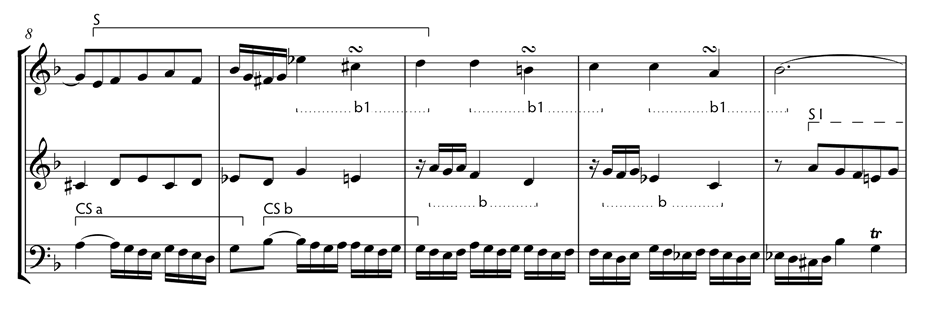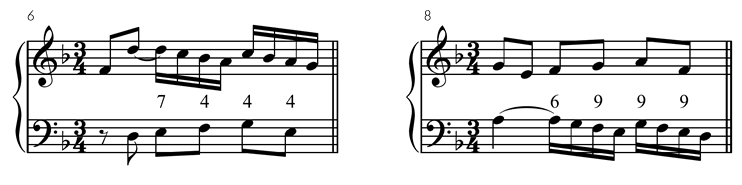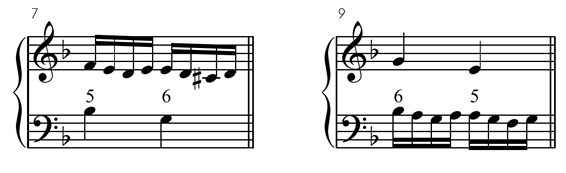First Episode

The first episode starts in measure 8 and ends in measure 12. Some interesting aspects in this episode:

• The subject (somewhat distorted) is presented by the soprano, beginning with a minor second and ending with a diminished third (a Neapolitan sixth chord, the Eb in the soprano).
• The subject is accompanied by the countersubject (in the bass). The countersubject uses inversion at the twelve (discussed in more detail below) in the first section (CS-a). The second section (CS-b) uses inversion at the eight. The original interval that joined the sections was a sixth, it is now a third and that is why the inversion interval changes.
• From measure 9 the alto doubles the soprano a lower sixth. The result is inversion at the tenth between alto and bass.
• The last notes of the subject are used on the next measures of the episode. Motive b1 in the soprano, motive b in the alto (changing the ascending sixth interval for a descending third) and the countersubject CS-b in the bass.
• In measure 12, the alto presents motive a transformed by inversion. It is in fact a false entrance.

Here is the discussed section:Let's take a closer look at the invertible counterpoint. The seventh interval in measure 6 becomes a sixth in measure 8. The fourth inverts into a ninth. This signals an inversion at the twelve as you can see in the inversion tables:Inversion at the twelve table

 This interval: 1 2 3 4 5 6 7 8 9 10 11 12 Inverts as: 12 11 10 9 8 7 6 5 4 3 2 1

Other methods to find the inversion type:

• Add the original interval and the inverted interval: 7 + 6 = 13
• Subtract 1: 13 - 1 = 12
• The result is the inversion type

Another method:

• Calculate the interval by which the top voice moves. In this case the D in measure 6 moves down to an A or a fourth.
• The E in the bass moves up to an F or a second
• We add both intervals: 4 + 2 = 6
• Subtract 1: 6 - 1 = 5
• 5 means inversion at the twelve (a twelve is a fifth plus an octave)

In measures 7 and 9 (bass and alto), the fifth interval inverts as sixth. This is inversion at the tenth:Inversion at the tenth table

 This interval: 1 2 3 4 5 6 7 8 9 10 Inverts as: 10 9 8 7 6 5 4 3 2 1